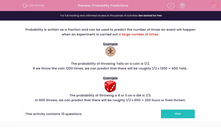# Use Probability to Predict How Often an Event Will Occur

In this worksheet, students will use probability to predict how often an event is likely to happen.This content is premium and exclusive to EdPlace subscribers.Key stage:  KS 2

Curriculum topic:   Maths and Numerical Reasoning

Curriculum subtopic:   Probability

Difficulty level:#### Worksheet Overview

Probability is usually written as a fraction and can be used to predict the number of times an event will happen when an experiment is carried out a large number of times.

Example 1The probability of throwing tails on a coin is 1/2.

If we throw the coin 1,200 times, we can predict that there will be roughly 1/2 x 1,200 = 600 tails.

Example 2The probability of throwing a 4 or 5 on a die is 1/3.

In 600 throws, we can predict that there will be roughly 1/3 x 600 = 200 fours or fives thrown.

Does that make sense?

Let's have a go at some questions now. If you want to look at this page again, just click on the red help button on the screen.

### What is EdPlace?

We're your National Curriculum aligned online education content provider helping each child succeed in English, maths and science from year 1 to GCSE. With an EdPlace account you’ll be able to track and measure progress, helping each child achieve their best. We build confidence and attainment by personalising each child’s learning at a level that suits them.

Get started